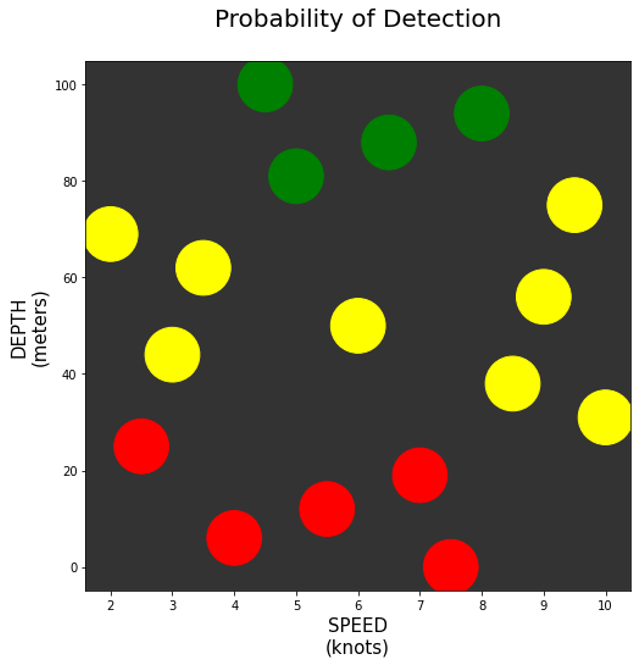## PyCubeDOE

Jataware, recently developed pycubedoe, a design of experiments module. Pycubedoe constructs a Nearly Orthogonal Latin Hypercube (NOLH) with user-defined factors and appropriate factor levels to design an experiment. Each experiment nearly eliminates correlation between the experiments which enables modelers to design ones that efficiently explore the available parameter space with as few model executions as possible.

#### Vignette

You are a submarine captain and wish to transit an area undetected. Only two factors determine whether or not you will be detected: speed (in knots) and depth (in meters). Your submarine is capable of making up to 10 knots underwater and can descend to a maximum depth of 100 meters.

You are provided with two models, probDetect_SPEED and probDetect_DEPTH, that predict the probability of detection for various speeds and depths.

What speed and depth should you make your transit?

First, bring your own model for the analysis. In this vignette, the detect model predicts the probability that at least one detection (due to speed or depth) occurs:

``````def detect(designPT):

speed = designPT
depth = designPT

#Calculate probability "at least one detection happens"
P_speed = 1 - probDetect_SPEED(speed)
P_depth = 1 - probDetect_DEPTH(depth)

P_detect = 1 - (P_speed * P_depth)
return P_detect``````

``````speed = [0,10,1]   # knots
depth = [0,100,0]  # meters

nums = {"speed":speed, "depth": depth}
cats = None

DOE = pc.pycubeDOE(nums,cats)``````

Then, run your model over each design point in the DOE:

``````modelResults = []
for designPT in pc.designPoints(DOE):
sim = detect(designPT)
modelResults.append(sim)``````

Finally, analyze the results. For this analysis, the captain told his analyst to categorize speed/depth pairings by their probability of detection: “low”=green, “medium” = yellow, and “high” = red:From the plot we can see to minimize the probability of detection, the captain should make his transit between 4 and 8 knots at a depth greater than 80 meters.